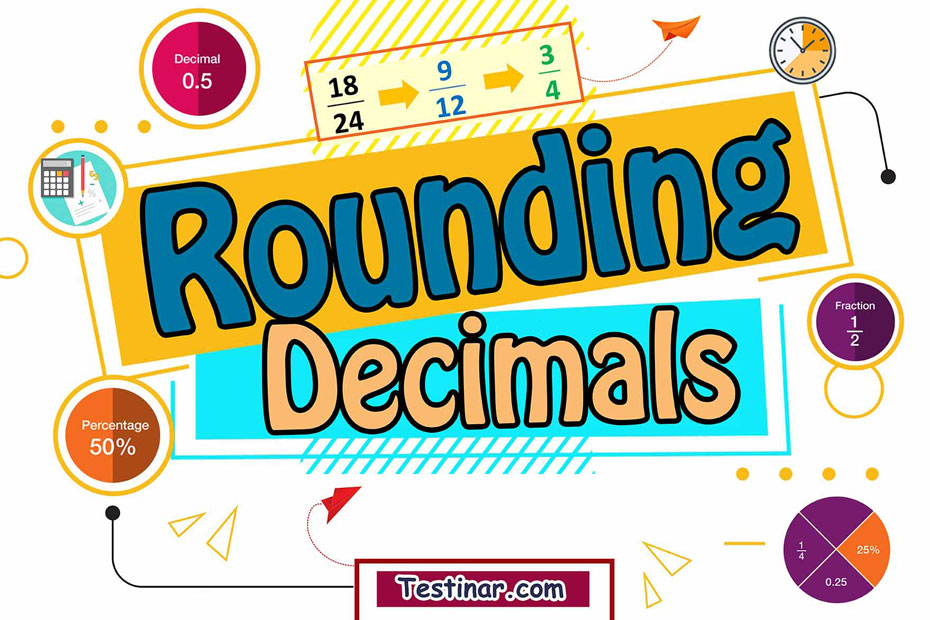## How to Round Off Decimals

In mathematics, a decimal can be defined as a number which has two parts: a whole part and a fractional part, and these two parts are separated by a decimal point. The whole part always represents a number greater than one, while the fractional part, i.e., the part after the decimal, always represents a number less than one.

For example, let’s take the number $$13.74$$. Now here the whole part is represented by $$13$$, whereas the fractional part $$(\frac{74}{100} \ )$$ is represented by $$74$$. Here $$74$$ can also be denoted as the decimal part, as it lies after the decimal point.

#### The Concept of Preceding Powers of 10

Now, there’s a very interesting concept linked to decimal numbers. This concept is known as the preceding powers of $$10$$. All decimal numbers are based on this concept. So, as we move from left to right in a decimal number, basically, the place value of every digit gets divided by $$10$$. So, the first digit after a decimal could be represented as$$\frac{1}{10}$$ , second as $$\frac{1}{100}$$ and so on.
So, from this concept, we can easily find out the expanded form of a decimal.

For example, let’s take the decimal number $$12.457.$$ The expanded form could be written as: $$10+2+ \frac{4}{10}+\frac{5}{100}+\frac{7}{1000}$$
Also, $$12.457$$ can be represented as $$12\frac{457}{1000}$$ in mixed fraction terms.

#### What is Rounding Off?

Rounding off is a process to estimate a number into its nearest accurate/approximate for. For example, $$12$$.395 can be completely rounded off to $$12$$, whereas $$12.678$$ can be rounded up to $$3$$.

#### How to Round Off Decimals

To round off a decimal, use the following steps:

• Take note of your place value. This is the number which is considered while rounding off.
• Now, look at the digit next to the place value. If this digit is greater than $$5$$, add 1 to the place value and remove all succeeding digits. If less than 5 then no change and remove all succeeding digits.

For example, $$2.67$$ becomes $$2.7$$,$$2.34$$ becomes $$2.3$$ and so on.

### Exercises for Rounding Decimals

1) $$3\underline{4}.697 \ \Rightarrow \$$

2) $$1\underline{4}.584 \ \Rightarrow \$$

3) $$11.\underline{4}05 \ \Rightarrow \$$

4) $$18.\underline{1}52 \ \Rightarrow \$$

5) $$41.496 \ \Rightarrow \$$

6) $$19.209 \ \Rightarrow \$$

7) $$12.332 \ \Rightarrow \$$

8) $$\underline{2}.971 \ \Rightarrow \$$

9) $$50.\underline{9}22 \ \Rightarrow \$$

10) $$57.\underline{1}25 \ \Rightarrow \$$

1) $$3\underline{4}.697 \ \Rightarrow \ \color{red}{35} \$$
First, look at the next place value to the right of the ones place. It’s $$6$$ and it is greater than $$5$$ , thus add $$1$$ to the digit in the ones place.
2) $$1\underline{4}.584 \ \Rightarrow \ \color{red}{15} \$$
First, look at the next place value to the right of the tenth place. It’s $$8$$ and it is greater than $$5$$ , thus add $$1$$ to the digit in the tenth place.
3) $$11.\underline{4}05 \ \Rightarrow \ \color{red}{11.4} \$$
First, look at the next place value to the right of the hundredth place. It’s $$0$$ and it is less than $$5$$ thus removing all the digits to the right. Then, the answer is $$11.4$$.
4) $$18.\underline{1}52 \ \Rightarrow \ \color{red}{18.2} \$$

5) $$41.496 \ \Rightarrow \ \color{red}{41} \$$

6) $$19.209 \ \Rightarrow \ \color{red}{19} \$$

7) $$12.332 \ \Rightarrow \ \color{red}{12} \$$

8) $$\underline{2}.971 \ \Rightarrow \ \color{red}{3} \$$

9) $$50.\underline{9}22 \ \Rightarrow \ \color{red}{50.9} \$$

10) $$57.\underline{1}25 \ \Rightarrow \ \color{red}{57.1} \$$

## Rounding Decimals Quiz

### ACT Math Ful Study Guide 2022 - 2023

$25.99$12.99

### CLEP College Algebra Study Guide 2020 – 2021

$20.99$15.99

### ParaPro Math Practice Workbook

$25.99$14.99

### CHSPE Math Practice Workbook

$25.99$14.99